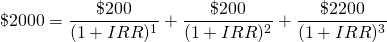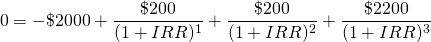# IRR formula

The internal rate of return (IRR) or effective rate of return is a number that is usually expressed as a percentage, and that measures the net return of an investment in a project. It is the rate of return that equates the present value of costs and benefits of the investment. A project can be considered interesting if the internal rate of return is high. On this page, we discuss how to calculate the internal rate of return using the IRR formula.

The internal rate of return is closely related to the required rate of return. In particular, the required rate of return is the minimum internal rate of return that the investor requires before he or she is willing to invest in a project.

## irr calculator

Mathematically, the internal rate of return can be determined be solving an equation that is a linear combination of powers equal to 1/ (1 + IRR). More specifically, the following formula can be used to solve for the IRR

It’s important to note that, often, the results cannot be calculated directly. This means that there’s no “closed form” solution to the problem. Instead, we need to solve the irr formula numerically. Fortunately, we can easily solve the irr formula problem using the ‘Solver’ in Excel. Alternatively, we can use the ‘XIRR’ function in Excel, which allows us to calculate the irr directly. First we provide an internal rate of return solved example.

## Internal rate of return example

Let’s consider the following example. Suppose we can invest \$2000 for a period of three years. Every year, we receive an interest payment ofTo solve this formula, we will typically rewrite the equation as followsThis makes it easier to solve the equation. In particular, we will try to determine the value of IRR for which we in fact arrive at zero. In practice, we will use software (e.g. Excel) to solve this formula numerically. The Excel file at the bottom of the page does exactly that.

## Summary

The internal rate of return is an important figure that used to evaluate the profitability of a project. Using Excel, we can create a simple IRR calculator. Alternatively, we can use the built-in function in Excel.

### Return calculation

Want to have an IRR implementation in Exel? Download the irr formula Excel file: IRR formula